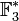# Transposition in symmetric group:S3

We consider the group$G$ defined as symmetric group:S3, i.e., the symmetric group of degree three, which for convenience we take to be the symmetric group acting on the set$\{ 1, 2, 3 \}$.

We are interested in the conjugacy class of transpositions in this group, i.e., permutations that flip two elements and fix the third element. The transpositions form a single conjugacy class and also form a single orbit under the action of the automorphism group of$S_3$.

The full list of elements in the conjugacy class is:$\{ (1,2), (2,3), (1,3) \}$

## Arithmetic functions

Function Value Explanation
order of the whole group 6
size of conjugacy class 3
size of automorphism class 3
number of conjugacy classes in automorphism class 1
order of elements in conjugacy class 2

## Description in alternative interpretations of the whole group

Interpretation of$G$ Description of conjugacy class Verification of size computation Verification of element order computation More information
As the symmetric group of degree three corresponds to the partition 2 + 1$\frac{3!}{(2)(1)} = 3$$\operatorname{lcm}\{ 2,1 \} = 2$ element structure of symmetric groups, element structure of symmetric group:S3#Interpretation as symmetric group, conjugacy class size formula in symmetric group
As the dihedral group$D_{2n}$,$n = 3, 2n = 6$ ($n$ odd) the conjugacy class of all "reflections" i.e. things outside the cyclic subgroup of index two$n = 3$ 2 element structure of dihedral groups, element structure of symmetric group:S3#Interpretation as dihedral group
As the special linear group$SL(2,q)$,$q = 2$ ($q$ even) the unique conjugacy class of unipotent elements (note that the conjugacy class is unique when$q$ is even)$q^2 - 1 = 2^2 - 1 = 3$ characteristic prime, i.e., prime whose power is$q$. In this case, it is 2 element structure of special linear group of degree two over a finite field, element structure of symmetric group:S3#Interpretation as special linear group of degree two
As the general affine group$GA(1,q)$,$q = 3$ the conjugacy class of all maps of the form$x \mapsto 2x + b, b \in \mathbb{F}_3$.$q = 3$ order of 2 in$\mathbb{F}_3^\ast$, which equals 2 element structure of general affine group of degree one over a finite field, element structure of symmetric group:S3#Interpretation as general affine group of degree one
As the general semilinear group$\Gamma L(1,p^2)$,$p = 2, p^2 = 4$ the conjugacy class of all maps whose field automorphism part is the non-identity map. Note that for generic$p$, there are$p - 1$ such conjugacy classes, but since$p = 2$, the conjugacy class is unique.$p + 1 = 2 + 1 = 3$ Since this is the conjugacy class containing the mapping that is simply the field automorphism, it has order 2. element structure of general semilinear group of degree one over a finite field, element structure of symmetric group:S3#Interpretation as general semilinear group of degree one